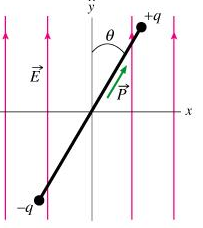# Problem: Consider an electric dipole located in a region with an electric field of magnitude E pointing in the positive y direction. The positive and negative ends of the dipole have charges +q and -q, respectively, and the two charges are a distance D apart. The dipole has moment of inertia I about its center of mass. The dipole is released from angle θ=θ0 and it is allowed to rotate freely. What is ωmax, the magnitude of the dipole's angular velocity when it is pointing along the y-axis?

###### FREE Expert Solution

Change in the electric potential:

ΔU = (Dq - Dqcosθ0)E = DqE - DqEcosθ0

Change in kinetic energy:

ΔK = (1/2)Iωmax2

In this situation, the potential and kinetic energies are equal. So we have:

(1/2)Iωmax2 = DqE - DqEcosθ0###### Problem Details

Consider an electric dipole located in a region with an electric field of magnitude E pointing in the positive y direction. The positive and negative ends of the dipole have charges +q and -q, respectively, and the two charges are a distance D apart. The dipole has moment of inertia I about its center of mass. The dipole is released from angle θ=θand it is allowed to rotate freely.

What is ωmax, the magnitude of the dipole's angular velocity when it is pointing along the y-axis?